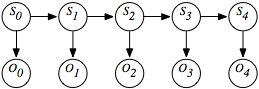6.5.2 Hidden Markov Models

A hidden Markov model (HMM) is an augmentation of the Markov chain to include observations. Just like the state transition of the Markov chain, an HMM also includes observations of the state. These observations can be partial in that different states can map to the same observation and noisy in that the same state can stochastically map to different observations at different times.

The assumptions behind an HMM are that the state at time t+1 only depends on the state at time t, as in the Markov chain. The observation at time t only depends on the state at time t. The observations are modeled using the variable Ot for each time t whose domain is the set of possible observations. The belief network representation of an HMM is depicted in Figure 6.14. Although the belief network is shown for four stages, it can proceed indefinitely.Figure 6.14: A hidden Markov model as a belief network

A stationary HMM includes the following probability distributions:

• P(S0) specifies initial conditions.
• P(St+1|St) specifies the dynamics.
• P(Ot|St) specifies the sensor model.

There are a number of tasks that are common for HMMs.

The problem of filtering or belief-state monitoring is to determine the current state based on the current and previous observations, namely to determine

P(Si|O0,...,Oi).

Note that all state and observation variables after Si are irrelevant because they are not observed and can be ignored when this conditional distribution is computed.

The problem of smoothing is to determine a state based on past and future observations. Suppose an agent has observed up to time k and wants to determine the state at time i for i<k; the smoothing problem is to determine

P(Si|O0,...,Ok).

All of the variables Si and Vi for i>k can be ignored.